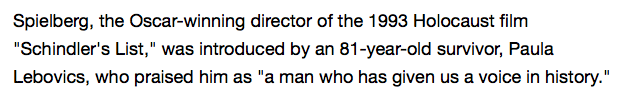## Monday, January 26, 2015

### 49 93 | Spielberg at Auschwitz, the Curious Timing of Holocaust Propaganda in 2015• '93 Film
• Spielberg = 19+16+9+5+12+2+5+18+7 = 93
Notice the name of the woman, and the age, who introduced Spielberg.
• Paula = 7+1+3+3+1 = 15
• Lebovics = 3+5+2+6+4+9+3+1 = 33
• Paula Lebovics = 48
• The number 81 has been of significance as of late
Let us close by looking at the curious Gematria of 'Auschwitz'.
• Auschwitz = 1+3+10+3+8+5+9+2+8 = 49
• Holocaust = 8+6+3+6+3+1+3+1+2 = 33
• Zyklon-B = 8+7+2+3+6+5+2 = 33
I recall how Saving Private Ryan was released in the time just before September 11, 2001, reenergizing those 'national war juices'.  For me, at the time, I felt like it was the closest I had ever come to understanding World War II.  Now I just look back and laugh.  That was also Spielberg's movie...  Now here he is doing a Holocaust Psy-Op in a time of major national insecurity.  Isn't it all too easy to see through?